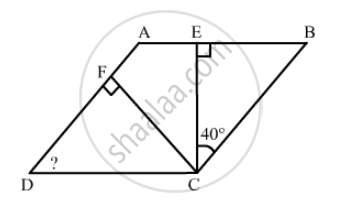Advertisement Remove all ads

# Find the angles marked with a question mark shown in Fig. 17.27 - Mathematics

Find the angles marked with a question mark shown in Fig. 17.27Advertisement Remove all ads

#### Solution

$\text{ In } \bigtriangleup CEB:$

$\angle ECB + \angle CBE + \angle BEC = 180° (\text{ angle sum property of a triangle })$

$40° + 90° + \angle EBC = 180°$

$\therefore \angle EBC = 50°$

$\text{ Also }, \angle EBC = \angle ADC = 50° \left( \text{ opposite angle of a parallelogram } \right)$

$\text{ In } \bigtriangleup FDC:$

$\angle FDC + \angle DCF + \angle CFD = 180°$

$50° + 90° + \angle DCF = 180°$

$\therefore \angle DCF = 40°$

$\text{ Now }, \angle BCE + \angle ECF + \angle FCD + \angle FDC = 180° (\text{ in a parallelogram, the sum of alternate angles is } 180° )$

$50° + 40° + \angle ECF + 40° = 180°$

$\angle ECF = 180° - 50° + 40° - 40° = 50°$

Is there an error in this question or solution?
Advertisement Remove all ads

#### APPEARS IN

RD Sharma Class 8 Maths
Chapter 17 Understanding Shapes-III (Special Types of Quadrilaterals)
Exercise 17.1 | Q 20 | Page 11
Advertisement Remove all ads

#### Video TutorialsVIEW ALL 

Advertisement Remove all ads
Share
Notifications

View all notifications

Forgot password?
Course